# 人工智能自动规划 09：无模型强化学习：Q-学习 和 SARSA

## 墨尔本大学 COMP90054 课程笔记

Posted by YEY on May 6, 2020

# Lecture 09 无模型强化学习：$Q$-学习 和 SARSA

1. 动机
2. 强化学习
3. $Q$-学习
4. SARSA
5. 总结

## 1. 动机

### 1.1 学习成果

1. 识别在哪些情况下，无模型强化学习适用于求解 MDP 问题。
2. 解释无模型规划与基于模型规划之间的差异。
3. 应用 $Q$-学习 和 SARSA 手动解决小规模 MDP 问题，并编写 $Q$-学习 和 SARSA 算法代码自动求解中等规模 MDP 问题。
4. 比较和对比非策略强化学习与策略强化学习。

### 1.2 规划与学习

• 搜索和价值/策略迭代都属于 基于模型 技术。这意味着我们需要知道模型；具体来说，我们知道 $P_a(s’\mid s)$ 和 $r(s,a,s’)$。

• $Q$-学习 和 SARSA 则属于 无模型 技术。这意味着我们不知道 $P_a(s’\mid s)$ 和 $r(s,a,s’)$。

• 如果我们不知道转移和回报，我们该如何计算策略呢？我们通过尝试行动并观察结果，从经验中学习，从而使它成为一个机器学习问题。

• 重要的是，在无模型强化学习中，我们不会尝试学习 $P_a(s’\mid s)$ 或 $r(s,a,s’)$ —— 我们将直接学习策略。

• 另外，有些技术则介于基于模型和无模型之间：基于模拟的技术。在这种情况下，我们将模型视为一个 模拟器，因此我们可以利用无模型技术来 模拟 $P_a(s’\mid s)$ 和 $r(s,a,s’)$ 并学习策略。

## 2. 强化学习

### 2.1 例子：神秘游戏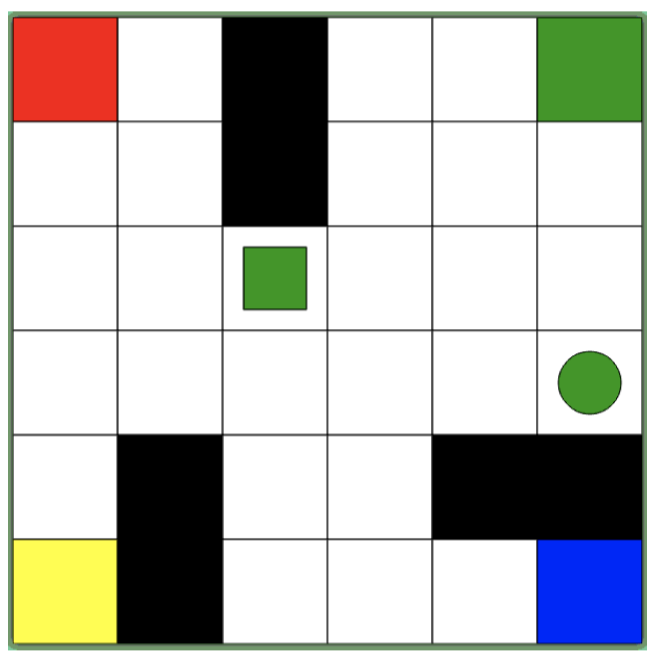• 你采取了什么程序？
• 你学到了什么？
• 你使用了什么假设？

$\to$ 想象一下，对于没有任何假设或直觉的计算机来说有多难！

• $1$：向上移动
• $2$：向左移动
• $3$：向右移动
• $4$：放下圆形色块
• $5$：向下移动
• $6$：拾取圆形色块

### 2.2 AI 规划和学习的方法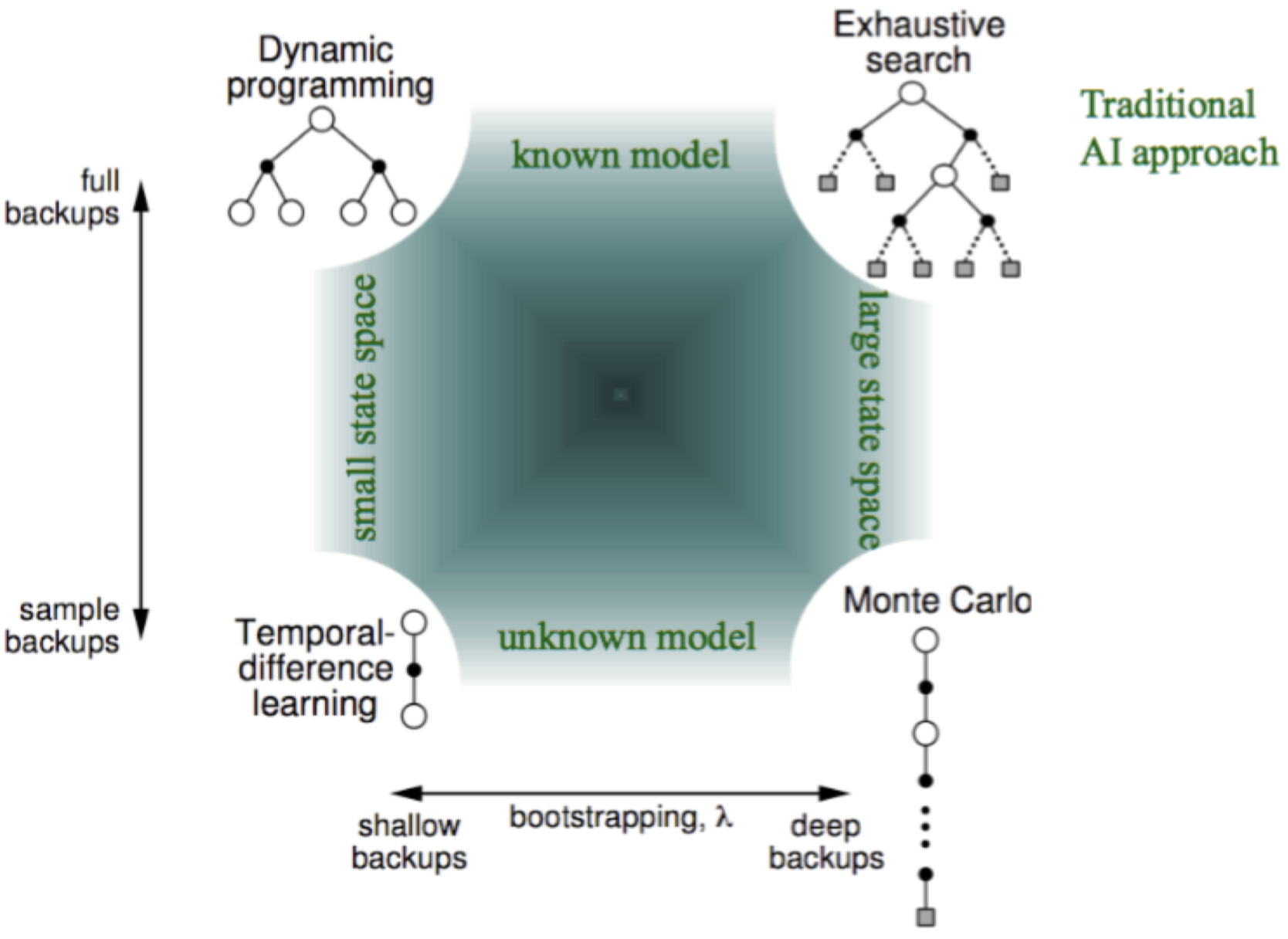### 2.3 强化学习：基础

• 我们对要解决的问题执行了许多不同的 episodes（注：agent 根据某个策略执行一系列行动到结束就是一个 episode），从中我们学习到一个 策略
• 在学习过程中，我们尝试学习在特定状态下应用特定行为的价值。
• 在每个 episode 中，我们需要执行一些行动。每次行动完成后，我们都会得到一个回报（可能为 $0$），并且我们可以看到新的状态。
• 由此，我们 强化（reinforce）了在先前状态下应用先前行动的估计。
• 我们在遇到以下情况时终止：（1）我们的训练时间用完了；（2）我们认为我们的策略已经收敛到了最优策略（对于每个新的 episode，我们不再看到任何改进）；或者（3）我们的策略已经 “足够好”（对于每个新的 episode，我们只能看到非常小的改进）。

### 2.3 基于模型 vs. 无模型

• 状态集合 $S$
• 转移概率 $P_a(s’\mid s)$
• 回报函数 $r(s,a,s’)$
• 折扣因子 $\gamma$

## 3. $Q$-学习

### 3.1 $Q$-学习算法

$Q$-学习 可能是最简单的强化学习方法，它的灵感源于动物如何从其周围环境中学习。这种直觉很简单直接。算法维护一个 $Q$-函数，该函数记录每个 “状态-动作” 对的 $Q(s,a)$。在每一步中：（1）使用多臂老虎机算法选择一个行动；（2）应用该行动并获得回报；（3）根据该回报更新 $Q(s,a)$。重复多次 episodes，直到……什么时候？

$Q$-学习算法：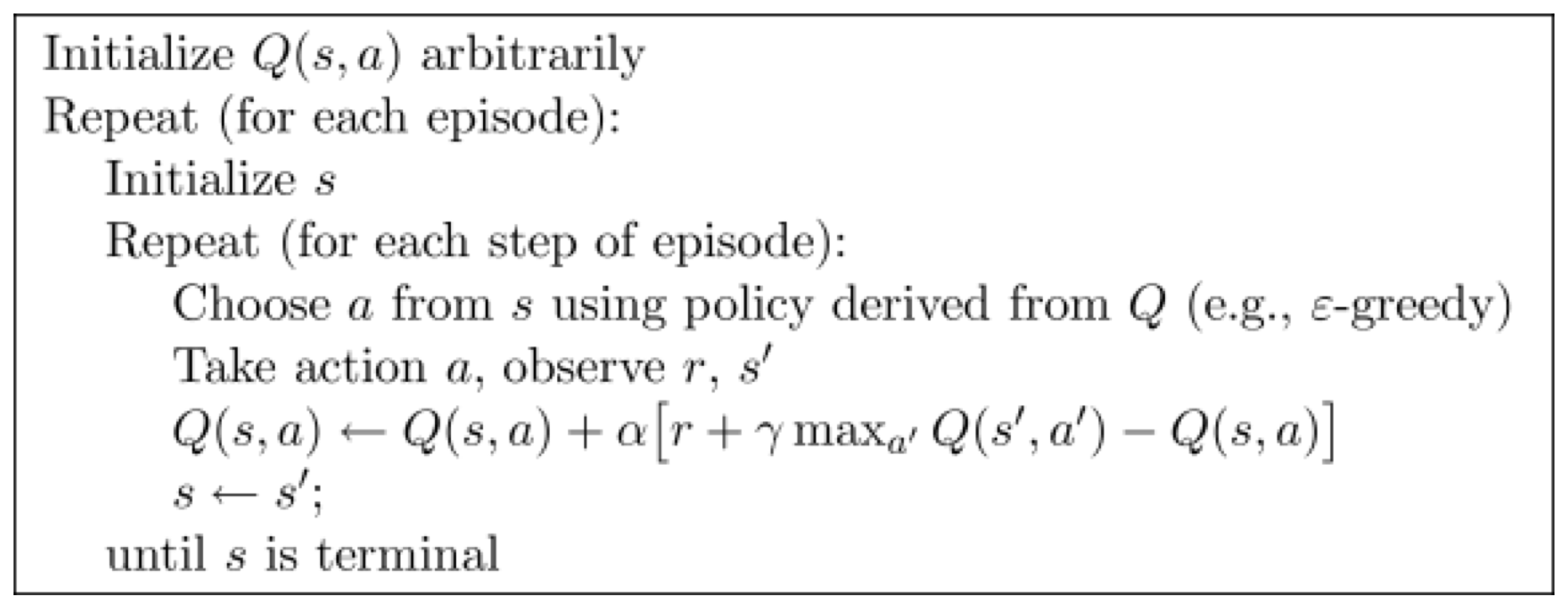### 3.2 更新 $Q$-函数

$Q$-函数 的更新发生在学习过程中（上面算法中的第 7 行）：

$Q(s,a) \leftarrow \underbrace{Q(s,a)}_{旧的值} + \underbrace{\alpha}_{学习率} \cdot [\overbrace{\underbrace{r}_{回报} + \underbrace{\gamma}_{折扣因子}\cdot \underbrace{\max_{a'}Q(s',a')}_{最优未来价值的估计}}^{估计回报} \underbrace{-Q(s,a)}_{不再计算额外的 Q(s,a)}]$

### 3.3 $Q$-函数：使用 $Q$-表

$Q$-表 是维护一个 $Q$-函数 的最简单的方法。它是一个表，其中包含了每个 $Q(s,a)$ 的条目。因此，就像价值迭代中的价值函数一样，它们不会缩放到较大的状态空间。（在下一讲中，将涉及更多有关缩放的信息）。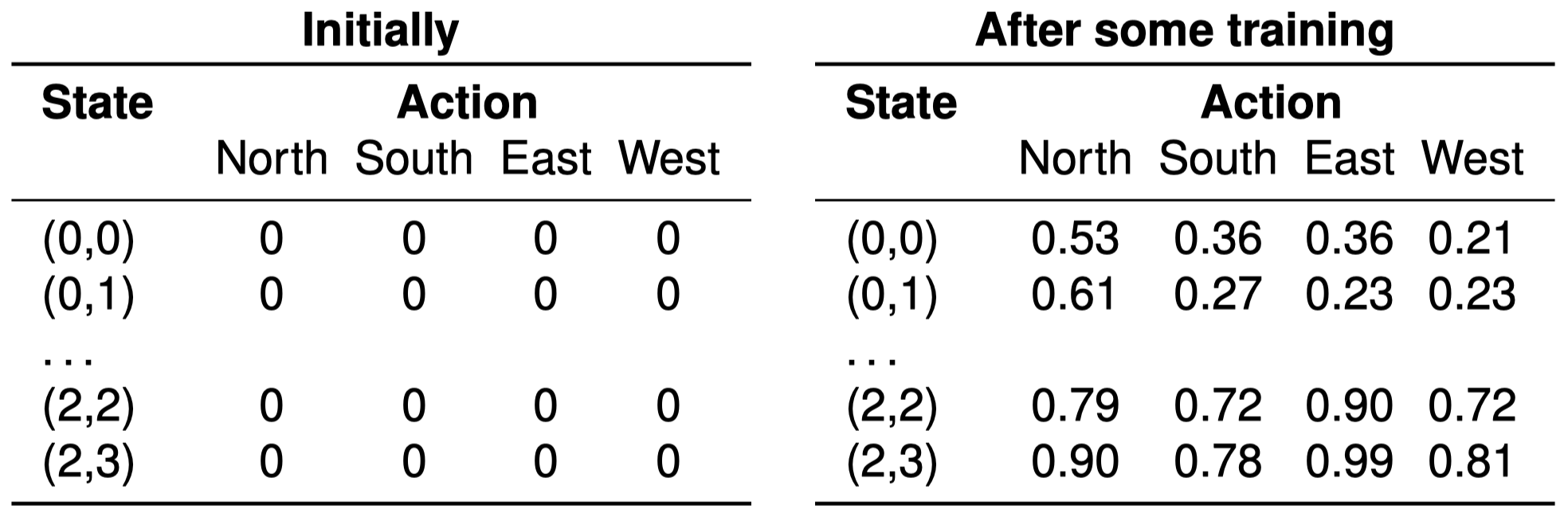### 3.4 $Q$-学习：例子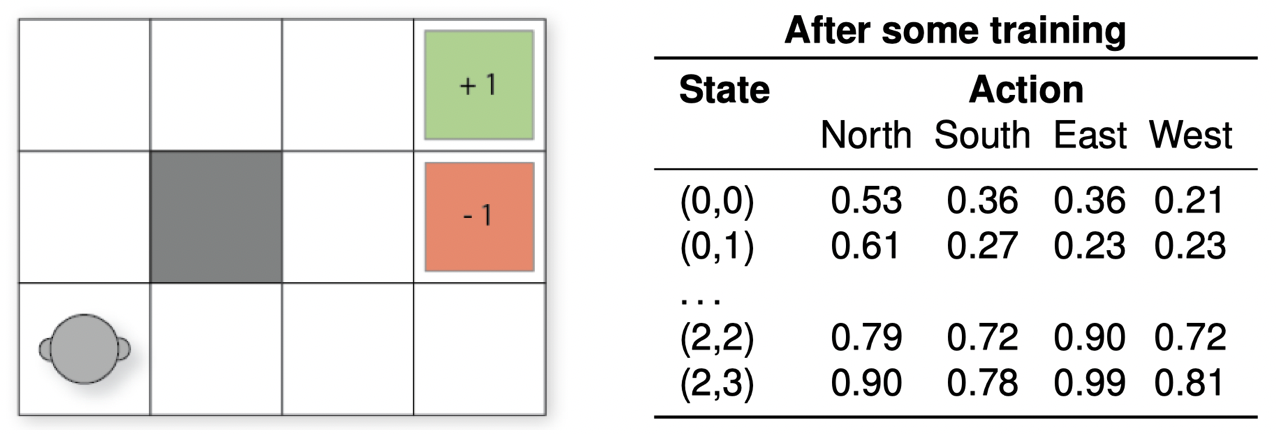\begin{align} Q((2,2),\text{North}) &\leftarrow Q((2,2),\text{North}) + \alpha[r + \gamma max_{a'}Q((2,2),a')-Q((2,2),\text{North})] \\ &\leftarrow 0.79+0.1\times[0 + 0.9\times Q((2,2),\text{East})-Q((2,2),\text{North})]\\ &\leftarrow 0.79+0.1\times[0 + 0.9\times 0.90-0.79]\\ &\leftarrow 0.792 \end{align}

### 3.5 利用 $Q$-函数

$\pi(s)=\mathop{\operatorname{arg\,max}}\limits_{a\in A(s)}Q(s,a)$

## 4. SARSA

### 4.1 SARSA：依附策略强化学习

SARSA $=$ State-action-reward-state-action

• 依附策略学习（On-policy learning）会对当前行为策略 $\pi$ 的 “状态-行动” 对 $Q^{\pi}(s,a)$ 进行估计，而 脱离策略学习（off-policy learning）在估计策略时会独立于当前行为。

SARSA 算法：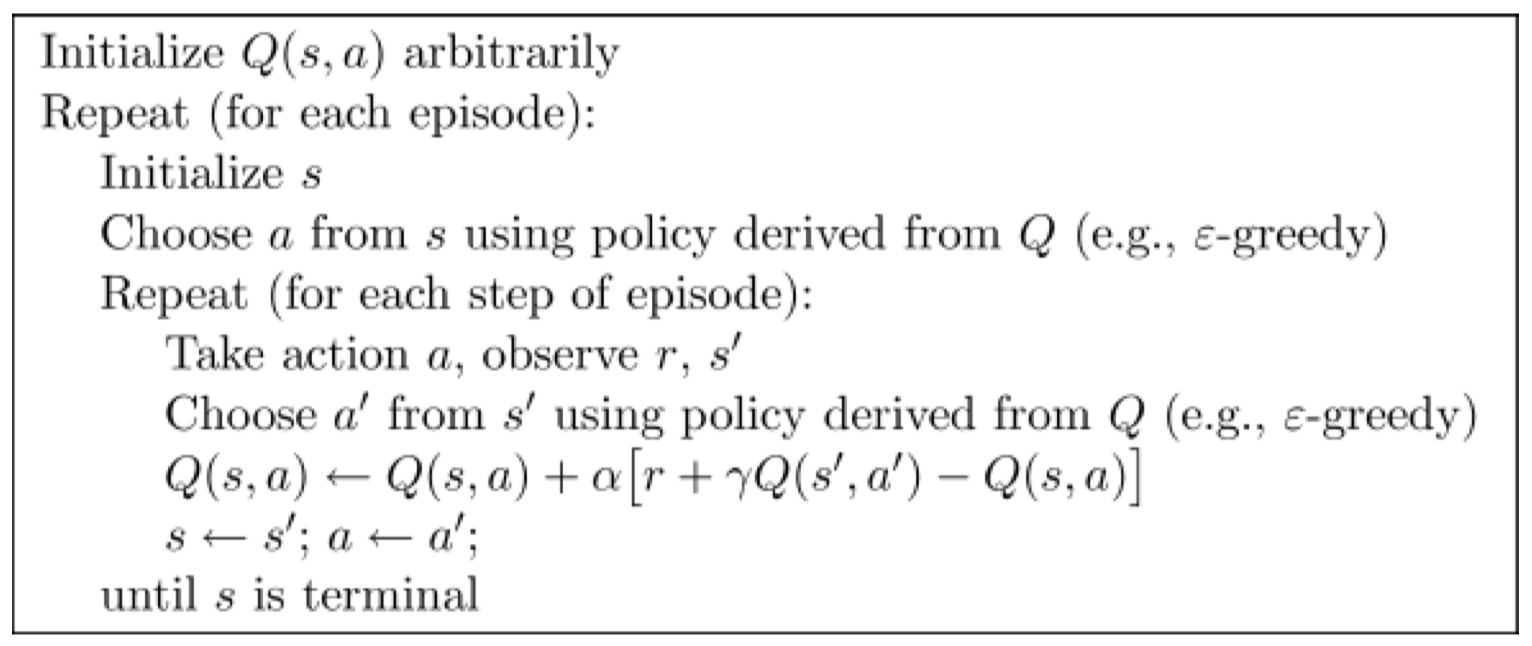### 4.2 $Q$-学习 vs. SARSA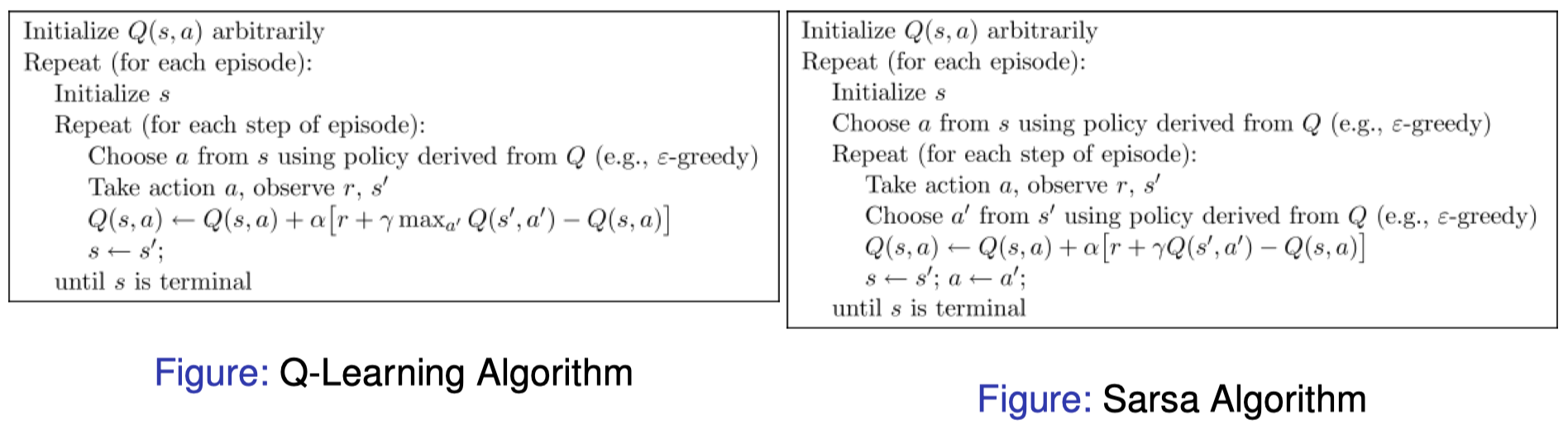### 4.3 依附策略 vs. 脱离策略：在这里有什么区别？

$Q$-学习：（1）选择一个行动 $a$；（2）执行该行动，并观察回报和下一个状态 $s’$；（3）通过假设未来回报是 $\max_{a’}Q(s’,a’)$ 乐观地 进行更新 —— 即，它假设未来行为是最优的（基于它的策略）。

SARSA：（1）为 下一次 循环迭代选择一个行动 $a’$；（2）在下一次迭代中，采取该行动，并观察回报和下一个状态 $s’$；（3）然后才为下一次迭代选择 $a’$；以及（4）使用估计的实际下一个行动（可能不是最贪婪的一个动作）进行更新（例如，可以对其进行选择以使其能够探索）。

### 4.4 SARSA：例子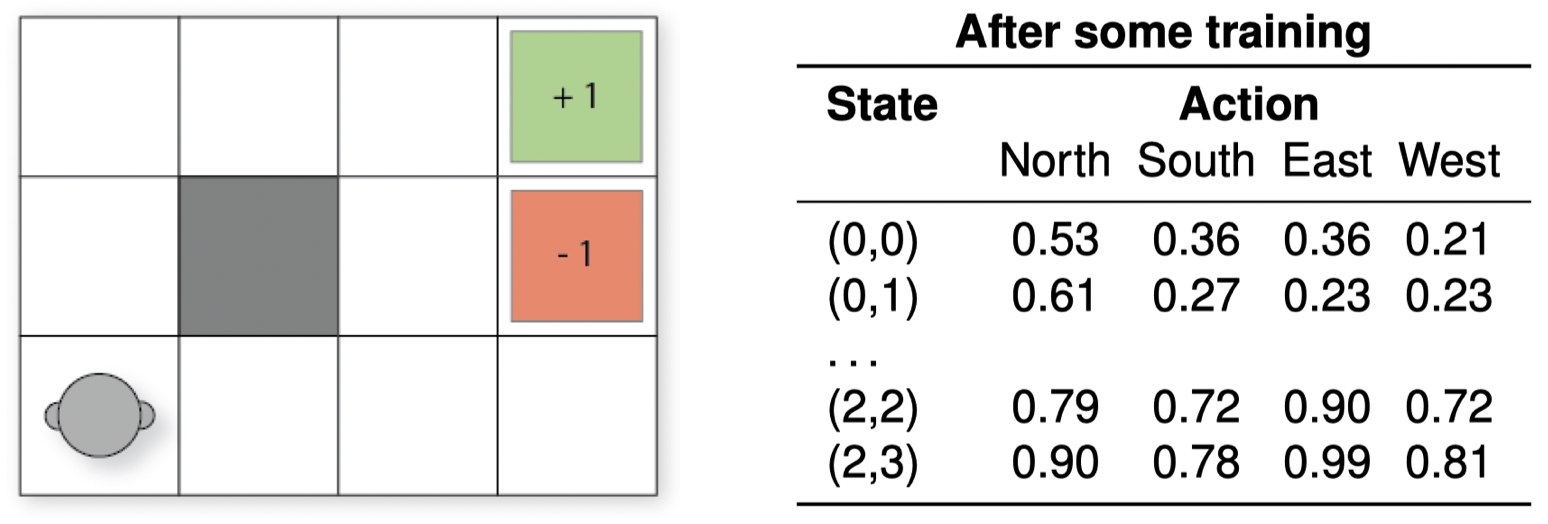\begin{align} Q((2,2),\text{North}) &\leftarrow Q((2,2),\text{North}) + \alpha[r + \gamma Q((2,2),\text{West})-Q((2,2),\text{North})] \\ &\leftarrow 0.79+0.1\times[0 + 0.9\times Q((2,2),\text{West})-Q((2,2),\text{North})]\\ &\leftarrow 0.79+0.1\times[0 + 0.9\times 0.72-0.79]\\ &\leftarrow 0.7758 \end{align}

### 4.3 依附策略 vs. 脱离策略：谁在乎？？

• $Q$-学习 将收敛到与所遵循的策略无关的最优策略，因为它是 脱离策略的：它在更新过程中使用贪婪回报估计，而不是遵循诸如 $\epsilon$-greedy 之类的策略。如果使用随机策略，$Q$-学习 仍将收敛到最优策略，但 SARSA 不会（或者说，不必要）。
• $Q$-学习 可以学习到一个最优策略，但这在 训练过程中 可能是 “不安全的” 或者冒险的。

### 4.4 SARSA vs. $Q$-学习：例子

$Q$-学习 沿着最优路径前进（沿着悬崖的边缘），但有时会由于 $\epsilon$-greedy 行动选择而跌落悬崖。SARSA 学习到的是安全路径，因为它是依附策略的，并且它在学习时会考虑选择行动的方法。下面右边的图显示了训练过程中 Sarsa 和 $Q$-学习 每次尝试的回报：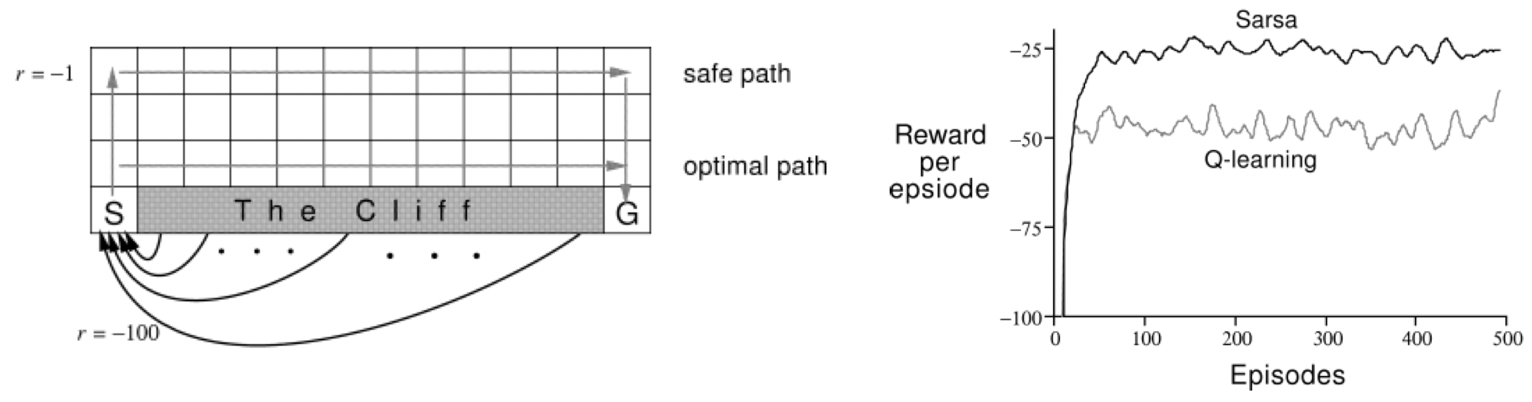SARSA 在 每次试验 中获得的平均回报要高于 $Q$-学习，因为它在以后的 episodes 中跌落悬崖的次数要少一些。但是，$Q$-学习 可以学习到最优策略。

### 4.5 依附策略 vs. 脱离策略：为什么我们会有这两种？

• 当我们要优化一个 在其环境中运行期间 进行学习的 agent 的行为时，依附策略学习更为合适。

我们将需要运行我们的云平台来获取数据。这样一来，如果使用依附策略能够让 每次试验 的平均回报更高，那么与脱离策略学习相比，它将给我们带来更好的总体结果，因为 “试验” 不是实践（实践表示实际上影响了我们赚多少钱）。

• 而当我们有足够的机会在 agent 正式投入运行之前对其进行训练时，脱离策略学习更为合适。

如果在部署之前，我们能够在模拟环境中运行我们的强化学习算法（并且我们有理由相信模拟环境是准确的），那么脱离策略学习效果会更好，因为它可以遵循其最优策略。

### 4.6 行动中的 $Q$-学习的例子

http://www.mnemstudio.org/path-finding-q-learning-tutorial.htm

## 5. 总结

• 离线：价值迭代、策略迭代。
• 在线：蒙特卡洛树搜索（MCTS）及其相关算法。

• 离线：强化学习
• 在线：蒙特卡洛树搜索（MCTS）及其相关算法。

• Checkers (Samuel, 1959)
首次将强化学习应用于有趣的真实游戏中
• (Inverted) Helicopter Flight (Ng et al. 2004)
表现超过人类
• Computer Go (AlphaGo 2016)
AlphaGo 以 4:1 击败前世界围棋冠军李世乭
• Atari 2600 Games (DQN & Blob-PROST 2015)
在超过 50 款游戏中，有半数以上达到人类玩家水准
• Robocup Soccer Teams (Stone & Veloso, Reidmiller et al.)
1999 年，世界最佳模拟足球运动员；2000 年，亚军
• Inventory Management (Van Roy, Bertsekas, Lee & Tsitsiklis)
在业界标准方法的基础上提升了 $10$-$15\%$
• Dynamic Channel Assignment (Singh & Bertsekas, Nie & Haykin)
世界上最佳的移动电话无线电频道分配者
• Elevator Control (Crites & Barto)
(可能是) 世界上最好的下行电梯控制器
• Many Robots
导航、双足行走、抓取、技能之间的切换……
• TD-Gammon and Jellyﬁsh (Tesauro, Dahl)
世界上最佳西洋双陆棋选手，宗师级水平。

## 6. 扩展阅读本作品采用知识共享署名-非商业性使用-相同方式共享 4.0 国际许可协议进行许可。 欢迎转载，并请注明来自：YEY 的博客 同时保持文章内容的完整和以上声明信息！